• Call Now

1800-102-2727•

# Vector Subtraction: Methods, Mathematical Formulation, Properties

When you swim perpendicular to the flow in a river from one bank to another bank then you will observe that you're not going the path of shortest distance. It is because of the relative velocity between you and the flow of the river.

Table of Content

## Negative of a Vector

A vector is said to be the negative of a given vector if it has the same magnitude and opposite in direction.

Suppose we have a given vector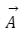, then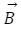be the negative of vector. and can be written as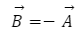Here we can see both the vectors have the same magnitude but opposite in direction.

## Subtraction of Vectors

Vector subtraction is the operation of finding the relative quantity of a vector with respect to other vector.

Suppose we have two vectorandthen subtraction of these vector is represented by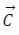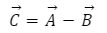Also, we can define subtraction of two vector in terms of addition of two vector as,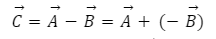Subtraction of two vectors is the addition of a vectorwith the negative of vectorFrom figure we can see negative ofis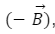, And resultant (Addition) ofandis represented by.

## Mathematical Formulation of Subtraction

If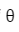is the angle between vectorandthen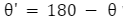will be the angle betweenandthen magnitude of vector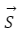can be find as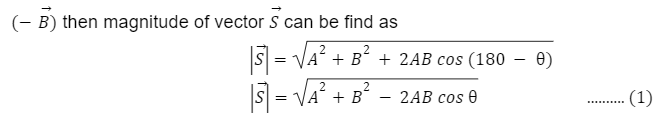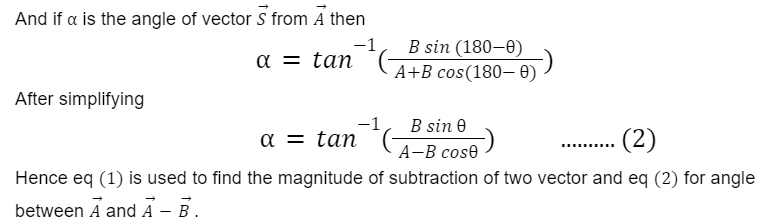## Properties of Subtraction of Vector

• Subtracting vectors is not commutative. This is because vector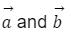are not the same (most of the time) and a negative sign affects a vector's direction.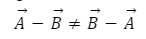• Similarly, subtracting vectors is not associative.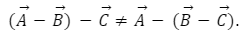.

•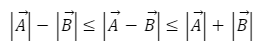## Practice Problems of Vector Subtraction

Question 1. Particle A is moving with the velocity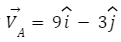and particle B is moving with velocity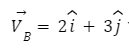find the velocity of A with respect to B.

Answer: Velocity of A with respect to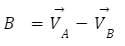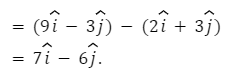Question 2. A boy is sailing a boat at night. He first sail 27.5 M in a direction 660north of east from his current location, and then travel 30.0 m in a direction 1120 north of east. If the boy makes a mistake and travels in the opposite direction for the second leg of the trip, where will he end up?

Answer: We can represent the first leg of the trip with a vector, and the second leg of the trip with a vector.Since the boy by mistake travels in the opposite direction for the second leg of the journey, the vector for the second leg of the trip will be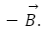. Therefore, he will end up at a location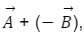or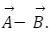.Note thatis of magnitude as B (30. 0 m), but in opposite direction,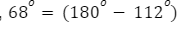south of east.

Draw the vectorsandand the resultant. Use ruler and protractor find  magnitude and direction of resultant vectorQuestion 3. Magnitude ofis 9 andis 12 and angle between them is 600.find the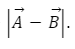.

Answer: From the subtraction of vector we know,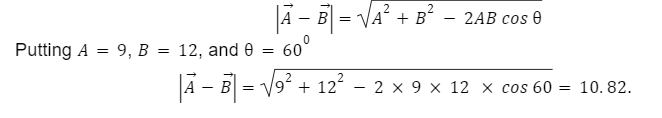Question 4. If Magnitude of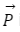is 7 and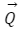is 5 and angle between them is 300.find the angle between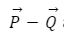and vector.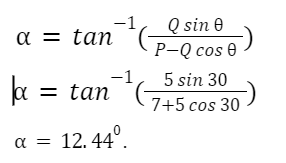## FAQs of Vector Subtraction

Question 1. What is negative of a vector?

Answer: Vector having the same magnitude and opposite in direction of a given vector is known as negative of a vector.

Question 2. What is the subtraction of a vector?

Answer: In general, It is addition of negative of vectorwith.

Question 3. What is the procedure of subtraction two vectors?

Answer: Keep two vectors tail to tail, now draw the resultant vector such that its head should be on the vector from which you are subtracting and tail on the vector which is subtracted.

Question 4. Is vector subtraction commutative.

## NCERT Class 11 Physics Chapters

 Physical World Units and Measurements Motion in a Straight Line Motion in a Plane Laws of Motion Work Energy and Power Particles and Rotational Motion Gravitation Mechanical Properties of Solids Mechanical Properties in Liquids Thermal Properties of Matter Thermodynamics Kinetic Theory Oscillations WavesTalk to our expert
Resend OTP Timer =
By submitting up, I agree to receive all the Whatsapp communication on my registered number and Aakash terms and conditions and privacy policy